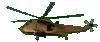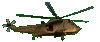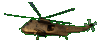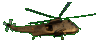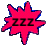Difference of Squares    Factoring      Fun Help     Game Tips:

- Perfect squares are formed by multiplying a quantity times itself, as in (3)(3) =9 .
Here are six perfect squares: 9,  25,  36,  42,  k2,  (y+3)2 .

- A 'Difference of Squares' expression is formed by subtracting one perfect square from another one.
Here are four 'Difference of Squares' expressions: 16-9,  49-r2,  k2-36,  (y+3)2-w2 .

- The Difference of Squares 16-9 can be factored into the two factors (4-3)(4+3).
If you check (4-3)(4+3) , it multiplies together to give you the value 7 which is the same as 16-9.

- The Difference of Squares 49-r2 can be factored as (7-r)(7+r).
If you check (7-r)(7+r) , it multiplies together to give you the original expression 49+7r-7r-r2 = 49-r2.
[To review multiplying a binomial times a binomial see the other SliderMath games.]

- As a general rule, the Difference of Squares a2-b2 can be factored as (a-b)(a+b).
You can use the two factors (a-b)(a+b) or you can use (a+b)(a-b). Both answers are equivalent.

- Your Score Report appears after you have made 10 choices.
- Your Game Score is reduced by the number of helicopter hits.

- To slow the game speed repeat tap/click on the word Slider.
- To increase the game speed repeat tap/click on the word Math.
- Speed can also be adjusted with a keyboard's - and + keys.

- Refresh/Reload the web page to restart the game.
- Adjust the sound level of media on your device.

More SliderMath